NCERT Solutions: Triangles (Exercise 7.3)

# Triangles (Exercise 7.3) NCERT Solutions - Mathematics (Maths) Class 9

Q1. ∆ ABC and ∆ DBC are two isosceles triangles on the same base BC and vertices A and D are on the same side of BC (see Fig). If AD is extended to intersect BC at P, show that
(i) ΔABD ≅ ΔACD
(ii) ΔABP ≅ ΔACP
(iii) AP bisects ∠A as well as ∠D.
(iv) AP is the perpendicular bisector of BC.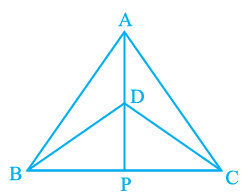Ans: In the above question, it is given that ΔABC and ΔDBC are two isosceles triangles.
(i) ΔABD and ΔACD are similar by SSS congruency because:
AB = AC (Since ΔABC is isosceles)
BD = CD (Since ΔDBC is isosceles)
∴ ΔABD ≅ ΔACD.
(ii) ΔABP and ΔACP are similar as:
AP = AP (It is the common side)
∠PAB = ∠PAC (by CPCT since ΔABD ≅ ΔACD)
AB = AC (Since ΔABC is isosceles)
So, ΔABP ≅ ΔACP by SAS congruency condition.
(iii) ∠PAB = ∠PAC by CPCT as ΔABD ≅ ΔACD.
AP bisects ∠A. — (i)
Also, ΔBPD and ΔCPD are similar by SSS congruency as
PD = PD (It is the common side)
BD = CD (Since ΔDBC is isosceles.)
BP = CP (by CPCT as ΔABP ≅ ΔACP)
So, ΔBPD ≅ ΔCPD.
Thus, ∠BDP = ∠CDP by CPCT. — (ii)
Now by comparing (i) and (ii) it can be said that AP bisects ∠A as well as ∠D.
(iv) ∠BPD = ∠CPD (by CPCT as ΔBPD ΔCPD)
and BP = CP — (i)
also,
∠BPD +∠CPD = 180° (Since BC is a straight line.)
⇒ 2∠BPD = 180°
⇒ ∠BPD = 90° —(ii)
Now, from equations (i) and (ii), it can be said that
AP is the perpendicular bisector of BC.

Q2. AD is an altitude of an isosceles triangle ABC in which AB = AC. Show that
Ans: It is given that AD is an altitude and AB = AC. The diagram is as follows: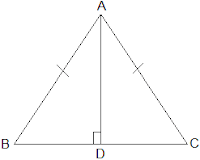(i) In ΔABD and ΔACD,

AB = AC (It is given in the question)
∴ ΔABD ≅ ΔACD by RHS congruence condition.
Now, by the rule of CPCT,
BD = CD.

Q3. Two sides AB and BC and median AM of one triangle ABC are respectively equal to sides PQ and QR and median PN of ΔPQR (see Fig). Show that:
(i) ΔABM  ΔPQN
(ii) ΔABC ≅ ΔPQR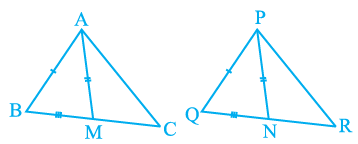Ans: Given parameters are:
AB = PQ,
BC = QR and
AM = PN
(i) ½ BC = BM and ½ QR = QN (Since AM and PN are medians)
Also, BC = QR
So, ½ BC = ½ QR
⇒ BM = QN
In ΔABM and ΔPQN,
AM = PN and AB = PQ (As given in the question)
∴ ΔABM ≅ ΔPQN by SSS congruency.
(ii) In ΔABC and ΔPQR,
AB = PQ and BC = QR (As given in the question)
∠ABC = ∠PQR (by CPCT)
So, ΔABC ≅ ΔPQR by SAS congruency.

Q4. BE and CF are two equal altitudes of a triangle ABC. Using RHS congruence rule, prove that the triangle ABC is isosceles.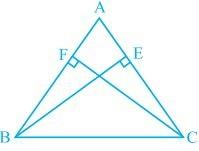Ans: It is known that BE and CF are two equal altitudes.
Now, in ΔBEC and ΔCFB,
∠BEC = ∠CFB = 90° (Same Altitudes)
BC = CB (Common side)
BE = CF (Common side)
So, ΔBEC ≅ ΔCFB by RHS congruence criterion.
Also, ∠C = ∠B (by CPCT)
Therefore, AB = AC as sides opposite to the equal angles is always equal.

Q5. ABC is an isosceles triangle with AB = AC. Draw AP ⊥ BC to show that ∠ B = ∠ C.
Ans: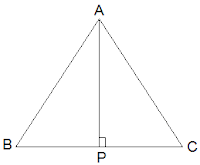In the question, it is given that AB = AC

Now, ΔABP and ΔACP are similar by RHS congruency as
∠APB = ∠APC = 90° (AP is altitude)
AB = AC (Given in the question)
AP = AP (Common side)
So, ΔABP ≅ ΔACP.
∴ ∠B = ∠C (by CPCT)

The document Triangles (Exercise 7.3) NCERT Solutions | Mathematics (Maths) Class 9 is a part of the Class 9 Course Mathematics (Maths) Class 9.
All you need of Class 9 at this link: Class 9

## FAQs on Triangles (Exercise 7.3) NCERT Solutions - Mathematics (Maths) Class 9

 1. What are the properties of a triangle?Ans. A triangle is a polygon with three sides and three angles. The properties of a triangle include the sum of its interior angles being equal to 180 degrees, the length of one side always being less than the sum of the lengths of the other two sides, and the difference between the lengths of any two sides being less than the length of the third side.
 2. How do you classify triangles based on their angles?Ans. Triangles can be classified based on their angles as acute, obtuse, or right triangles. An acute triangle has all three angles measuring less than 90 degrees, an obtuse triangle has one angle measuring greater than 90 degrees, and a right triangle has one angle measuring exactly 90 degrees.
 3. What is the Pythagorean theorem and how is it used in triangles?Ans. The Pythagorean theorem states that in a right triangle, the square of the length of the hypotenuse (the side opposite the right angle) is equal to the sum of the squares of the lengths of the other two sides. This theorem is commonly used to find the length of one side of a right triangle if the lengths of the other two sides are known.
 4. Can a triangle have two right angles?Ans. No, a triangle cannot have two right angles. The sum of the interior angles of a triangle is always 180 degrees, and if two angles are right angles, their sum would be 180 degrees, leaving no room for a third angle.
 5. How can we determine if two triangles are similar?Ans. Two triangles are similar if their corresponding angles are congruent and the ratios of the lengths of their corresponding sides are equal. This can be determined using the angle-angle (AA) similarity criterion or the side-side-side (SSS) similarity criterion.

## Mathematics (Maths) Class 9

62 videos|438 docs|102 tests

## Mathematics (Maths) Class 9

62 videos|438 docs|102 tests
Signup to see your scores go up within 7 days! Learn & Practice with 1000+ FREE Notes, Videos & Tests.
10M+ students study on EduRev
Track your progress, build streaks, highlight & save important lessons and more!(Scan QR code)
Related Searches

,

,

,

,

,

,

,

,

,

,

,

,

,

,

,

,

,

,

,

,

,

;